# Bihar Board 12th Physics Important Questions Short Answer Type Part 2

BSEB Bihar Board 12th Physics Important Questions Short Answer Type Part 2 are the best resource for students which helps in revision.

## Bihar Board 12th Physics Important Questions Short Answer Type Part 2

Question 1.
How the range of a voltmeter increased?
Let us suppose that the resistance of a voltmeter is v and its range is v volts. We are to increase its range from ν volts to ν1 volts (ν1 > ν).

In order to increase the range of a voltmeter a resistance of r1Ω is connected in series with it so that when a potential difference of ν1 volts is applied between the ends of the voltmeter and the free end of r1 that much current flows through it that the pointer attached in the voltmeter wives a full scale deflection.

For a voltmeter reading up to volts only the current required for the full scale deflection is given by
I = $$\frac{v}{r}$$ ………….(i)

When a resistance of r1Ω is connected in series with the voltmeter the total resistance is now r + r1. In this position the current required for the full scale deflection in the voltmeter will be given by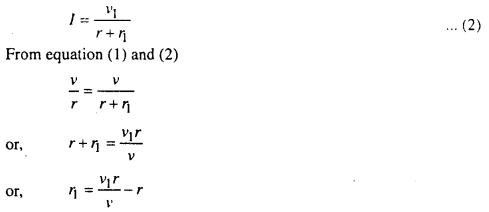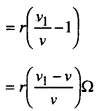Hence a resistance equal to $$r\left(\frac{v_{1}-v}{v}\right)$$ ohm will be required to be connected in series with the voltmeter.

Question 2.
Why do you always connect a ammeter in series and voltmeter in parallel?
Ammeter is used to determine the current passing through any circuit. It is joined in series so that total current passing through the circuit passes through it. Resistance of the ammeter is also very small so that total resistance and current in the circuit with ammeter remains nearly the same as total current and resistance of the circuit without ammeter.

Voltmeter is used to measure the potential difference of circuit. Therefore voltmeter is connected in parallel to that part of circuit which potential difference has to be calculated. Resistance of the voltmeter is large, so that current passing through this equipment is very small.

Question 3.
Describe Oersted experiment.
Oersted in his experiment noticed the magnetic effect of electric current. In his experiment, a wire AB is kept above a magnetic needle NS. When current flows in the wire, magnetic needle gets deflected. Here needle tries to be perpendicular to the wire. When direction of the current is reversed, the deflection is also reversed.From this experiment Oersted came to the conclusion that there is some dap relation between the electric current and magnet.

Question 4.
What do you understand by electrical work energy and power?
Or, Show that watt = volt × ampere.
Let a current c be passed through a wire in t sec. The potential difference is produced at the ends of the wire.
Amount of work done = charge × potential diff.
= (current × time) × potential diff.
= c.t.v = vct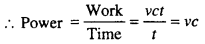Taking unit of all quantities
Watt = Volt × Ampere

Question 5.
What is the difference between A.C. and D.C.?

• D.C. flows in one direction and its value is constant where as the value of A.C. changes periodically with time and hence it has positive and negative values.
• A.C. can be transferred from one place to other with less loss of energy but D.C. cannot transferred.
• A.C. can be controlled by choke coil with least loss of energy where as D.C. is controlled only by ohmic resistance in which there is maximum loss of energy.
• The machines depend on A.C. have longer life in comparision to the machine which depend on D.C.
• A.C. is more dangerous than D.C. because the people feel more shock with A.C.

Question 6.
Derive in expression for forces between two parallel current carrying conductors.
M and N are two conductors placed parallel to each other and separated by a distance d. They carry currents l1 and l2. Conductor M will produce a field of induction B1 at all near by points.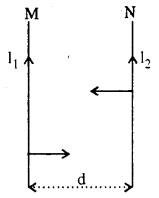∴ Magnetic field produced by current l1 at any point of N is
B1 = $$\frac{\mu_{0} I_{1}}{2 \pi d}$$
This field acts prependicular to N and into the plane of paper. It exerts a force on N carrying current I2. Hence force exerted on N is
F = B1I2l where ‘l’ is the length of the conductor.
= $$\frac{\mu_{0} I_{1} I_{2} \cdot l}{2 \pi d}$$ [Putting the value of B1]

If the currents are in same direction, this force acts on N towards M. similarly. Conductors N also exerts an equal force M towards itself. Hence the two wires get attracted towards each other.

Question 7.
Explain the Definition of ampere.
Force between two parallel current carrying conductors
= $$\frac{\mu_{0}}{2 \pi} \frac{I_{1} I_{2}}{d}$$
where I1 & I2 → currents
l → length
d → distance between conductors.
If I1I2 = 1 ampere, l = 1 metre
and d = 1 metre then
F = $$\frac{\mu_{0}}{2 \pi}=\frac{4 \pi \times 10^{-7}}{2 \pi}$$
i.e. = 2 × 10-7 NewtonHence one ampere current is that current flowing through two straight conductors of length one metre each and one metre apart produces between then a force of = 2 × 10-7 newton

Question 8.
How can convert the galvanometer into voltmeter?
A galvenometer is converted into a voltmeter by connecting a high resistance in series. The scale is calibrated into volts.Let resistance of galvanometer = G
High resistance = R
Current = I
Potential difference between A and B
= current × resistance
i.e. ν = I(g + R)
or, g + R = $$\frac{v}{I}$$
or, R = $$\frac{v}{I}-g$$
This high resistance $$\frac{v}{I}-g$$ connected in series changes galvanometer into voltmeter.

Question 9.
How can convert galvanometer into ammeter?
To convert galvanometer into an ammeter, a low resistance is connected in parallel. This low resistance is called shunt. The scale is calibrated into amperes.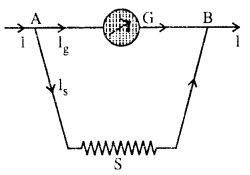Let resistance of Galv = G
Total current = I
Current through Galv = Ig
Current through shunt = Is
At point A, I = Ig + Is ………..(1)
Potential diff, between A and B
= Current. Resistance
= Ig · G
= Is · S
IgG = Is, S
IgG = (I – Ig)s [From Eq. (1)]
or, S = $$\frac{I g \cdot G}{I-I g}$$
Thus low difference $$\frac{I g \cdot G}{I-I g}$$ connected in parallel changes galv. into ammeter.

Question 10.
What is current density?
Current density is defined as the amount of current upon per unit area.
It is denoted by J
current density = $$\frac{\text { Current }}{\text { Area }}$$
J = $$\frac{I}{A}$$
Its unit is A/m2
Its dimension is IL-2

Question 11.
What is mobility?
It is defined as the drift velocity per unit electric field.
It is denoted by µ.
∴ mobility = $$\frac{\text { Drift velocity }}{\text { Electric field }}$$
µ = $$\frac{v_{d}}{E}$$
It’s Unit is m2/ν – S

Question 12.
What is drift velocity?
Drift velocity is the average uniform velocity acquired by free electrons inside a metal by the application of an electric field which is responsible for current through it.
It is denoted by νd.
Let a conductor of length ‘l’ and cross sectional area is ‘A’
volume of conductor = Al
If n be a number of free electrons in unit to volume then
Number of free electrons in conductor = nAleQuestion 13.
Obtained the expression for energy of conductor?
The work done in charging uncharged conductor is known as energy of conductor.
Let a conductor of capacitance C is given an electric charged q to raise its potential through zero to v.
∴ Average potential = $$\frac{0+v}{2}=\frac{v}{2}$$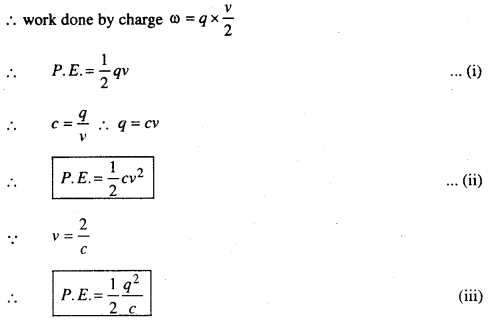Equation (i), (ii) and (iii) are the expression for Energy of conduction.

Question 14.
What do you mean by colour code of carbon resistance?
It is a method for determination of the resistance of electronics devices. In this method determined the resistance by help of colour bands of code 0, 1, 2 … 9. In this method used ten colours. The band of first and second colour are represented the significant numbers, third band in indicates the decimal multiplier and forth band indicates the tolerance of the resistor. In this method used colours are black, brown, red, orange, yellow, green, blue, voilet, Grey and white.

Question 15.
What is Eddy current.
When a mass of metal, is placed in changing magnetic field, induced currents were produced resulting in the dissipation of energy in the form of heat. These are called Eddy current.

In order words that induced currents are circular in nature is called Eddy current.

Question 16.
Defined surface density?
The amount of charge per unit area is known as surface density.
It is denoted by σ
surface density = $$\frac{\text { charge }}{\text { area }}$$
σ = $$\frac{q}{A}$$
Its unit is c/m2

Question 17.
Define para-magnetic substance?
That materials which when placed in magnetic field acquire a weak magnetisation in the same sense as the applied magnetic field is called paramagnetic substance, it’s magnetic susceptibility is very small and positive.
Ex.: O2, Mn, Cr, Al, Pt etc.

Question 18.
Define Diamagnetic substance?
That materials which when placed in a story megnetic field acquire a weak magnetisation in a direction opposite to that of the applied magnetic field is called diamagnetic substance. It’s magnetic susceptibility is negative.
Ex.: Bi, Ag, H2O, H2 etc.

Question 19.
Define dielectric strength and dielectric constant for relative permitivity.
Dielectric strength: The maximum electric field that a dielectric medium can with stand without breaking down of its insulting property is called the dielectric strength, for different permittive material, dielectric strength is different. For dry air at normal pressure, dielectric strenght is about 3 × 106 Vm-1.

Dielectric constant (or relative permittivity): It is defined as the ratio of the permittivity of the medium to the permittivity of the free space. It is denoted by ∈r or k.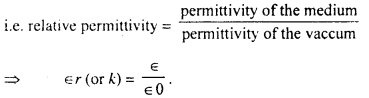It has nor unit and dimension being the ratio of two similar quantities. For free space or air ∈r = 1 (taken)

Question 20.
What is choke coil?
A choke coil is an electrical appliance used for controlling current in an a.c circuit. It works on the principle of wattless current. The choke coil consists of a coil wounded over a laminated shoft iron core. The inductive reactance offered by the coil XL = ωL is large. Therefore current is reduced
iv = $$\frac{e_{v}}{X_{L}}$$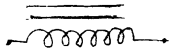For an ideal choke coil, ohmic resistnace is zero. Therefore, energy loss is zero and current lags behing the applied voltage by 90°. So Power consumed is 0. For low freq. a.c. current; iron core choke is in to increase the value of XL = ωL = 2πνL and is called a.f. choke. But for high freq. ac. air choke is used. This is called r.f. choke.

Question 21.
Why core of transformer is made laminated?
When a.c. current flows through the coils of transformer, the magnetic flux linked with the core is also changing with time and eddy current produced in it which produces heat in it. This is also known as iron loss. To prevent or minimise it core of transformer is made in the form of insulated striped core. In laminated core eddy current is not formed and loss of electric current is minimised.

Question 22.
What is Lana’s law?
Lenz’s law: The direction of the induced current or e.m.f. is known by lens’s law. According to which “The polarity of induced emf or the direction of induced current is such that it opposes the change in magnetic flux responsibile for the its production.”

For example, in fig. with north pole of a bar magnet is being pushed towards the coil. The amount of mag. flux linked with coil increases. The current is induced in the coil in such a direction it opposes the increage in flux. This possible only when current induced in the coil is in anticlockwise with respect to an observer.Lenz’s law is applicable in all situation of varying mangetic flield (a fluxed) linked with a coil. Lenz’s law obey the priciple of conservation of energy and also says about the source of induced emf.

Question 23.
What do you mean by self induction and coefficient of self induction?
A current flows through a coil B. Due to this, a magnetic field is set up. If the current is changed, the total flux changes and an induced e.m.f. is set up in the current.

Thus in the coil due to its own current the production of e.m.f. is called self induction.
If I be the current flowing through the coil and the flux produced is Φ then
Φ ∝ I
= LI

Where L is a constant called coefficient of self induction on self inductance.
If I = 1
then L = Φ
Hence self induction is the flux produced in the coil by unit current in it.
Its unit is “Henry”.

Question 24.
An induced e.m.f, has no direction of its own comment?
Yes an induced e.m.f. has no direction of its own. The direction depends upon the change in magnetic flux because in accordance with Lenz’s law, the induced current always opposes the change in magnetic flux.

Question 25.
What do you mean by mutual induction and coefficient of mutual induction?
In this figure P and S are two will P is called primary coil and S is called secondary coil. In the P circuit, there is a battery B and a key K. In the S circuit there is a galvanometer.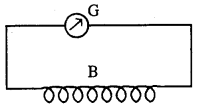When the key K is closed the current flows in P. A momentary current produced in S. On switching off the key K, again a momentary current produced in 5 in the reverse direction. Thus due to current in P, e.m.f. is. produced in S. This phenomena is called “Mutual induction”.If I be the current through P and Φ be magnetic flux produced in S then
Φ ∝ MI
= MI

Where M is a constant called coefficient of mutual induction or mutual inductance.
If I = 1 amp then M = Φ
Hence the magnetic flux produced in the secondary coil by passing unit current in primary coil is called coefficient of mutual induction.
Its unit is “Henry”.

Question 26.
What do you mean by wattless current?
Let us consider an A.C. current which has either inductive coil or condense. The value of its ohmic resistance is zero. In this case phase difference between current and voltage is 90°.
Hence loss of average power
= Vrms Irms·cos90°
Where Vrms and Irms are r.m.s. voltage and current.
= Vrms · Irms · 0
= 0

From above, it is clear that current is flowing in the circuit but the average power of the circuit is zero.
This current is called “wattless current”.

Question 27.
Soft iron is used in electromagnets but steel for permanent magnet Why?
Soft iron has a very high magnetic susceptibility so it can be magnetised very easily in an external magnetic field. But steel has a very high resistivity. So when a steel rod is magnetised it remains the magnetism developed in it even after the removal of the external magnetic field. But soft iron loses its magnetism. When the external magentic field is removed in electromagnets we need very strong magnetic field.

So when soft iron is used it exhibits strong magnetic field so long as the electric current flows through the coil of the electromagnet. In the case of a permanent magnet, steel is used as it is required that magnetism in it should be present for a long time. Also steel has a high coercivity and due to this property of steel even rough handling of it does not effect its magnetism.

This is why soft iron is used for making electromagnets and steel for permanent magnets.

Question 28.
How can it be tested experimentally whether a given liquid is paramagnetic or diamagnetic?
When a paramagnetic material is kept in a magnetic field it tends to stronger field but when a diamagnetic material is subjected to such a field it tends to move from the stronger to the weaker field.

For experimental test the given liquid (for test) is taken a watch glass. The watch glass (with the small quantity of liquid kept in it) is put on the two pole pieces of a strong electromagnet which are very close to each other. When a current is passed in the electromagnet circuit, either the liquid (in the watch glass) shows an elevation in the middle or shows a depression in the middle. In the former case the liquids under test is a paramagnetic liquid while in the latter case it is a diamagnetic liquid.

Question 29.
Expression for Torque on a magnetic dipole (bar magnet in a uniform magnetic field).
Let SN be a magnet. Its pole strength is and magnetic length is 2l. It is placed in a uniform magnetic field H. The magnet makes an angle θ with field H.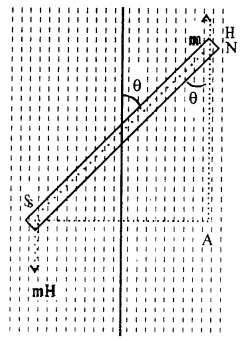The force acting on N and S pole = mH.
There two forces are equal, paralled and act at the two ends of a magnet and so constitute a couple.
Torque or moment of the couple (C)
= Force × perpendicular distance between the two forcesHence magnetic moment of a magnet is defined as the couple acting on a magnet placed at right angles to a uniform magnetic field of unit strength.

Question 30.
Explain the elements of the earth’s magnetism or magnetic elements.
The quantities which determine the magnitude and direction regarding earth’s magnetism are called “magnetic elements”.

The magnetic elements are three in number:

• Declination
• Dip or inclination
• Horizontal component.(i) Declination: The angle between the magnetic meridian and geographical meridian is called “declination”.
In fig. SANE is magnetic meridian and SCDB is geographical meridian. The angle θ between them gives the declination i.e. ∠ASC = θ.

(ii) Dip: The angle between the direction of total earth’s magnetic intensity and horizontal line in the magnetic meridian is known as “angle of dip” or “angle of inclination”.

In fig. SN is the total earth’s magnetic intensity expressed by I. SA is the horizontal line in the mag. Meridian expressed by H.
The angle between I and H is called dip (δ)
Hence H = I cos δ

(iii) Horizontal component: At a place the total magnetic field due to earth has a horizontal component on the meridian called horizontal field(H).
Hence ∠ASN = δ

Question 31.
What do you understand by Nuclear fission and nuclear fusion?
Nuclear fission: In 1938 Otto Hahn and Strassman discovered that when uranium was bombarded with neutrons, it split up into two fragment- barium (z = 56) and krypton (z = 36). It was also found that enormous energy is released. This process is called “Nuclear fission”.
92U235 + 0n156Ba141 + 36Kr92 + 30n1 + energy
In this fission average 3 neutrons are produced.
These neutrons cause fission of other nuclei of U235 and thus chain reaction starts.Nuclear Fusion: Nuclear reaction that proceed at very high temperature (10°C) are called nuclear fusion. In these reactions enormous energy is liberated.

Question 32.
Do x-rays and γ-rays have the same nature of origin?
x-rays and γ-rays do not have the same nature of origin. Production of .x-rays and γ-rays are of two types-

1. Continuous x-rays and
2. Characteristics x-rays.

The production (origin) of continuous x-rays are explained as follows – When cathode rays are incident on a target and penetrated well inside the atom, then electron from the higher energy orbits jumps to fill in the gaps of the K and M orbits. The difference in the energy of the electrons of both the orbits is manifested in the form of characteristics x-rays, production of γ-rays. The energy of a stable nucleus is minimum and is then said to be in the ground state. It can be excited by bombarding particles or photons of higher energy. When an excited nucleus to its ground state, γ-rays are produced.

Question 33.
What is photo electric effect?
Initially, this effect was invented by a telephone operator W. Smith on 1885. Hertz approved it in 1887. According to Hertz electrons are emitted when light of very short wavelength falls of a metal. In 1988, Halwachs found that a zinc plate if negatively charged lost its charge when it was illuminated by ultraviolet.

In 1900, Leonard and J.J. Thomson proved experimentally that electrons are emitted when light of suitable wavelength falls upon it. These electrons are called “Photo Electron.” These electron moves towards +ve plate. So electric current is called “Photo electric current.” This phenomena is called “photo electric effect.”

Question 34.
What is work function? What is threshold frequency?
Work Function: According to Einstein, when light of frequency v is incident on a metallic surface, photons of energy hν strikes the metal. A photon gives its energy hv to the electron and the electron is ejected out of the metal. If a photon ejects a photo electron of velocity ν then
hυ = $$\frac{1}{2}$$mν2 + Φ

Where Φ is the work done (or the energy spent) in just bringing the electron outside the surface and is known as work function so the energy required to release an electron just out of the surface of the metal is called the work function of the metal concerned. It depends upon the nature of the metal.

Threshold frequency: For each metal there is a certain minimum frequency of the incident radiation called the threshold frequency below which no photo electron is emitted from the surface of the metal.

Question 35.
What is difference between conductor, semi conduction and insulator with respect to energy gap?

1. There is no energy gap between conduction band and valence band in conductor.
2. There is very small (= 1 eν) energy gap between conduction band and valence band in semi-conductor.
3. There is very large (= 10 eν) energy gap between conduction band and valence band in insulator.

Question 36.
What are n-type and p-type semi-conductors?
Semi-conductors have resistivity between 10-1Ωm to 10-4Ωm. Antimony (Sb) is pentavalent while germanium (Ge) is tetravalent semi-conductor.

(i) n-type semi-conductor- If antimony is added to germanium and a careful controlled heating and ring process is allowed to be conducted, the Sb atoms will replace Ge ions at many places in the lattice. When an Sb atoms is symmetrically surrounded by four Ge atoms, the Ge atoms will form a covalent bond with four Sb ions leaving the fifth Sb atom free. In this way there are a number of free electrons.

The energy of this extra electron is slightly less than that as the bottom of the conduction band as 0.01 eV of energy is needed to detach this excess fifth electron from the atom. The excess electrons increase the conductivity of Ge. This type of impurity as that of Sb producing extra electrons is called donor. The doped Ge semi-conductor is known as the n-type semi-conductors, the electrons being the current carriers as a means of negative current carriers.

(ii) P-type semi-conductor: If a trivalent impurity like iridium is added to Ge (or silicon) then one iridium atom replaces one Ge atom in the crystal lattice. The three iridium electrons form covalent band with the three of the four Ge electrons, leaving a vacancy, called hole, for one electron. The energy level of the positive hole is just above the filled valency band. The electron from this valency band excited by the thermal agitation fills up the hold leaving a vacancy (hole) in its place. The conductivity of the semi-conductor increases due to the positive hole conduction. The impurity added is acceptor as it is capable of accepting electrons. Because of the positive charge carriers the semi-conductor is known as p-type.

In the case of intrinsic semi-conductors, the current carriers holes and electrons are equal. In the n-type semi-conductor, electrons are majority carrier while the positive holes are minority carriers in the p-type semi-conductors, the holes are majority carriers and the electrons minority carriers.

Question 37.
Explain how a p-n junction can be used as a diode?
We imagine a semi-conductor one part of which is p-type and other one is n-type. AB is the divider. This is called p-n junction n-type contains more electron, so it moves to the other part to fill up hole and some +ve hole of p-type moves this side to collect electron. This reduces +ve charge on the p-type and electron on n-type. Due to this a layer of charge forms at the junction and causes a potential differences. This stop electron and +ve hole to cross barrier.
This potential is called Barrier potential.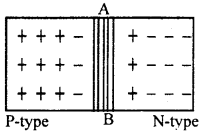If p-type of p-n Jn. is joined to +ve pole of cell and n-type to -ve, then this connection is called “Forward bias connection.” But if p-type to -ve and n-type to +ve then this is called “Reverse bias connection.”

A.C. can be transferred to D.C. by p-n Jn. When p-n Jn. is forward biased then effect of electric double layer not allowing charge carriers to cross the Jn. is reduced hence current flows. The current increases as larger potential difference is applied by the battery.

On the other hand, when p-n Jn. is reversed biased then effect of electric double layer is not allowing charge carriers to cross the Jn. is supported by the battery. Hence no current flows.
Thus p – n Jn. acts as a diode.

Question 38.
What is diode valve? Why is it called a “valve”?
Diode valve was made by Fleming in 1904. It consists of two elements-

1. Filament
2. Plate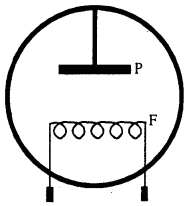That’s why it is called ‘Diode’. Its filament F is attached in series to battery. Plate P is attached to ‘+ve‘ electrode and filament F is attached to ‘-ve’ electrode.

When Electric current flows, F becomes warm and emits electron. It is attracted towards P plate. Due to this, electric flows.

If plats P is attached to -ve electrode, then it repulses the electron emitted from F.
That’s why, no current passes. Therefore current passes only when P is attached to ‘+ve’ charged electrode and hence it is called ‘valve’. valve is used to allow current to pass through one direction only.

Question 39.
What is isotopes?
Thomson found in his experiment that two types of atom had the same chemical properties. Properties called these two types of atom as “isotopes” Thus isotopes are defined as-

“The atoms of an element having the same atomic number and identical chemical properties but different atomic weight are called the isotopes of that element.”

For example oxygen has three isotopes represent by 8H16, 8O17, 8O18 having atomic weight 16, 17, 18 respectively. These three isotopes have the same atomic no. z = 8.

Hydrogen has three isotopes namely hydrogen (1H2), deuterium (1H2) and tritium (1H1).

Question 40.
What do you understand by De-Broglie wave?
Light has a properties of photon and wave. Hence light has a dual character. The wave length of these waves depend upon the momentum which in turn depends upon the mass and the velocity:

These waves are called “matter wave” or “De-Broglie wave”.
Let υ be the frequency of light wave and h be the Planck’s Constant then energy of photon = hυ ……..(1)
According to Einstein theory of relativity,
Energy = mc2 …………….(2)
Where is the velocity of light.
Comparing eq. (1) and eq. (2), we get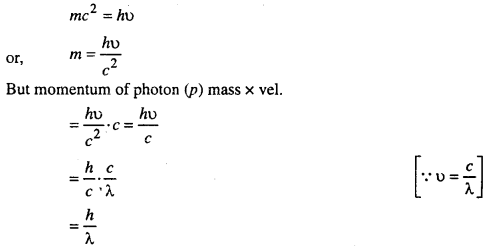or, λ = $$\frac{h}{p}$$  ………….(3)
De-Broglie suggested that a moving particle is associated with a wave. If m be the mass and ν be the velocity of the particle, momentum (p) = mν
Hence from eq. (3),
λ = $$\frac{h}{m v}$$
This is known as De-Broglie wave equation.

Question 41.
What is transistor?
A transistor is a semi-conductor device corresponding to a thermionic vacuum triode. It can amplify electric signal and can also be used as an oscillator. In its original form, it was invented by John Bardeen and waiter house Brattain in 1948. An improved form was devided by william Bradford. Transistors operate at much lower power and are better than electronic tuber in many respects. These are widely used in portable radio and television receivers.

Question 42.
What is junction transistors?
These are basically two types of transistors- (i) Point contact, (ii) Junction transistor. But junction transistors are more in use compared to point type transistors. They are preferred due to their ruggedness and small size.

Junction transistors are classified into two types PNP and NPN. Thus the junction transistor is an extention of the junction diode and is analogous to the triode valve. It has three electrodes called emitter base and collector.

PNP-type is formed by joining a thin layer of N-type germanium between two thick layer of P-type.NPN type: It is formed by joining a thin layer of P-type between two thick layer of N-type.Question 43.
What is Boolean Algebra?
Boolean algebra is a system of mathematics based on logic with its own set of fundamental laws. George Boole gave this algebra to represent logical statement. By using Boolean logic, we can reduce almost all problems to a series of questions which can be answered by “Yes” or No and can be represented by 1 or 0.

Boolean algebra is based on three base-
(i) OR (ii) AND (iii) NOT
There are called “Boolean Operator”.

Question 44.
State the properties of electro magnetic waves.
(i) Electro magnetic waves are produced by accelerated charges.
(ii) Electro magnetic waves propagate in the form of varying electric and magnetic fields. The fields are not only perpendicular to each other but also to the direction of propagation of wave. Due to this reason, the electro magnetic waves are transverse waves.
(iii) Electro magnetic waves do not require a material medium for their propagation.
(iv) The velocity of e.m. waves in free space is equal to the velocity of light in free space.
(v) Speed of e.m. waves the free space is given by
C = $$\frac{1}{\sqrt{\mu_{0} \epsilon_{0}}}$$
Where µ0 and ∈0 are the permeability and permittivity of free space.
But in a material medium,
Speed (C) = $$\frac{1}{\sqrt{\mu \in}}$$
Where µ and ∈ are the absolute permeability and permittivity of the medium.
(vi) Electro magnetic waves obey the principle of super position.

Question 45.
Discuss the spectrum of electro magnetic radiation.
Electro magnetic spectrum is the orderly distribution of electro-magnetic radiations in accordance with their wave length or frequency. The usual classification is below-
(i) Radio waves: These waves have wave length ranging from a few kilo meter down to 0.3m. The frequency range is from a few Hz to 109 Hz. These waves are used in television and radio broadcasting.

(ii) Micro waves: These waves have wave length ranging from 0.3m down to 10-3 m. The frequency range is from 109 Hz upto 3 × 1011 Hz. These waves are used in radar and other communication systems.

(iii) Infra red spectrum: This covers wave length from 10-3 m down to 7.8 × 107m. The frequency range is from 3 × 1011 Hz upto 4 × 104 Hz. These waves have many application in industry, medicine etc.

(iv) Optical spectrum: This covers wave length from 7.8 × 10-7 m down to 3.8 × 10-7m and frequencial from 4 × 1014 Hz upto 8 × 1014 Hz optics (light) has so many uses.

(v) Ultraviolet rays: This covers wave length from 3.8 × 10-7 m down to nearly 6 × 10-10 m with frequency from 8 × 1014 Hz to nearly 3 × 1017 Hz. These rays are used in some medical applications and also in sterilisation processes.

(vi) x-rays: These rays have wave length from nearly 10-9 down to wave lengths of nearly 6 × 10-12 m and frequencies between 3 × 1017 Hz and 5 × 1019 Hz. x-rays are used in medical diagnosis.

(vii) Gamma rays: This covers wave length 10-10 to 10-14 m and frequencies between 3 × 108 Hz and 3 × 1022 Hz.

Question 46.
Why sky waves are not used in the transmission of TV signals?
When the frequency is above 40 MHz, the ionosphere is unable to reflect the signals back to Earth. TV signals have a frequency range of 100 to 200 MHz. So the transmission of TV signals via the sky wave is not possible.

Question 47.
Convert decimal number 25·8125 into binary number.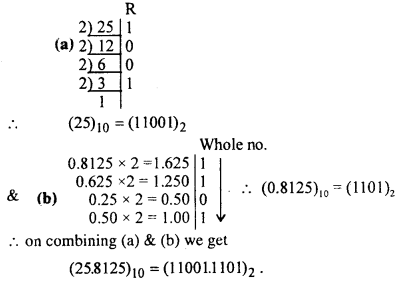The plasma frequency at the peak of a layer (ionosphere) is called critical frequency (Vc) and for reflection from the ionosphere the value of Vc is given by Vc = 9$$\sqrt{N}$$.